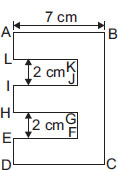# User Forum

Subject :IMO    Class : Class 5

In the given figure, DE = HI = AL = 2 cm and LK = IJ = HG = EF = 5 cm. Find the perimeter of the given figure (in cm).A 52
B 34
C 54
D 70

## Ans 1:

Class : Class 5
AL=2,KJ=2,IH=2,GF=2,ED=2.SO,2 2 2 2 2=10.BC=10so10 10=20breadth=7x2=14so20 14=34how can option C be correct

Class : Class 7